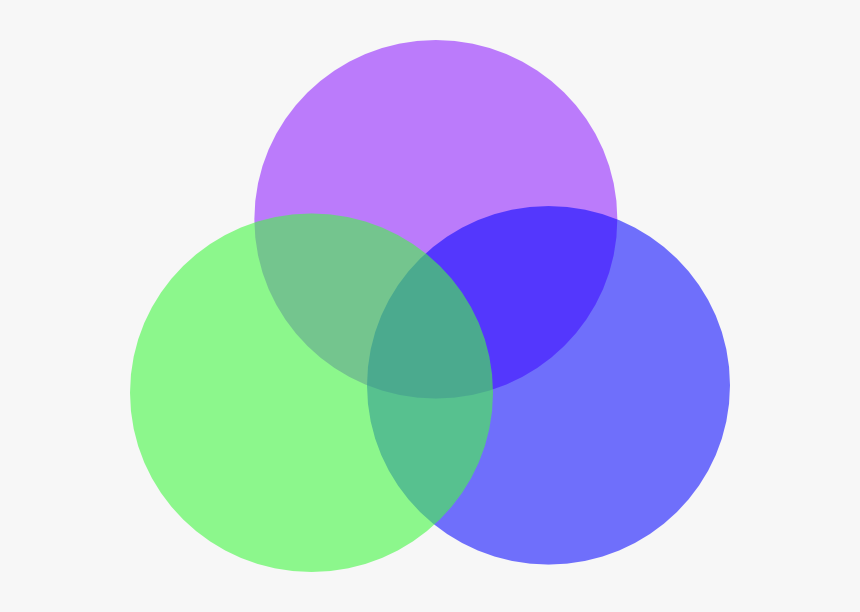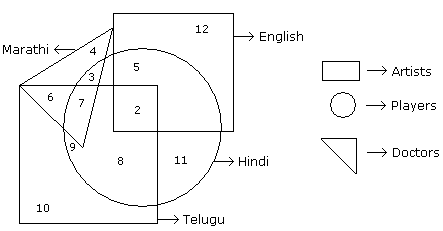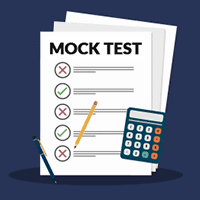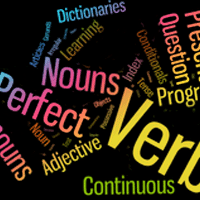Courses

# Venn Diagrams - Introduction and Examples (with Solutions) Quant Notes | EduRev

## CAT: Venn Diagrams - Introduction and Examples (with Solutions) Quant Notes | EduRev

The document Venn Diagrams - Introduction and Examples (with Solutions) Quant Notes | EduRev is a part of the CAT Course IBPS Clerk Prelims - Study Material, Mock Tests.
All you need of CAT at this link: CAT

What is a Venn Diagram?

• A Venn diagram (also called a set diagram or logic diagram) is a diagram that shows all possible logical relations between a finite collection of different sets.Venn Diagram
• Typically overlapping shapes, usually circles, are used, and an area-proportional or scaled Venn diagram is one in which the area of the shape is proportional to the number of elements it contains.
• These diagrams represent elements as points in the plane and sets as regions inside curves. An element is in a set S just in case the corresponding point is in the region for S. They are thus a special case of Euler diagrams, which do not necessarily show all relations.
• Venn diagrams were conceived around 1880 by John Venn. They are used to teach elementary set theory, as well as illustrate simple set relationships in probability, logic, statistics, linguistics and computer science.
Examples
• In the following figure, the small square represents the persons who know English, the triangle represents those who know Marathi, the big square represents those who know Telugu and the circle represents those who know Hindi.
• In the figure, different regions from 1 to 12 are given.Q1. How many persons can speak English and Hindi both languages only?
A. 5
B. 8
C. 7
D. 18

• Number of persons who can speak English and Hindi both only is 5.

Q2. How many persons can speak Marathi and Telugu both?

A. 10

B. 11

C. 13

D. None of these

6 + 7 = 13.

Q3. How many persons can speak only English?
A. 9
B. 12
C. 7
D. 19

• Number of persons were can speak English is 12.

Q4. How many persons can speak English, Hindi and Telugu?
A. 8
B. 2
C. 7
D. None of these

• Number of persons who can speak English, Hindi and Telugu is 2.

Q5. How many persons can speak all the languages?
A. 1
B. 8
C. 2
D. None

• There is no such person who can speak all the languages.
The document Venn Diagrams - Introduction and Examples (with Solutions) Quant Notes | EduRev is a part of the CAT Course IBPS Clerk Prelims - Study Material, Mock Tests.
All you need of CAT at this link: CATUse Code STAYHOME200 and get INR 200 additional OFF Use Coupon Code
All Tests, Videos & Notes of CAT: CAT

### Top Courses for CAT## IBPS Clerk Prelims - Study Material, Mock Tests

73 videos|43 docs|81 tests

### Top Courses for CATTrack your progress, build streaks, highlight & save important lessons and more!

,

,

,

,

,

,

,

,

,

,

,

,

,

,

,

,

,

,

,

,

,

;